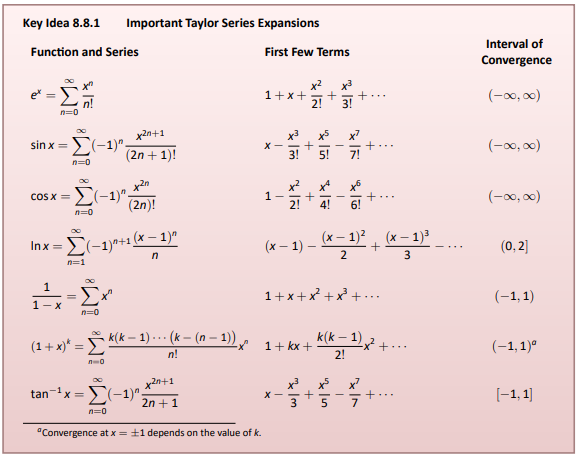## Calculus Discussion 14

### Question 4

Key Idea 8.8.1 gives the nth term of the Taylor series of common functions. Verify the formula given in the Key Idea by finding the first few terms of the Taylor series of the given functions and identifying a pattern.Taylor Series

The following is the formula for a Taylor series for function f(x).

$f(x) = f(a) + f'(a)(x-a) + \frac{f''(a)}{2!}(x-a)^2 +...+ \frac{f^n(a)}{n!}(x-a)^n$

#### Derivatives

f(x) = sin(x), c=0

• f(0) = sin(0) = 0
• f’(x) = cos(x) -> cos(0) = 1
• f’’(x) = -sin(x) -> -sin(0) = 0
• f’’’(x) = -cos(x) -> -cos(0) = -1
• f’’’’(x) = sin(x) -> sin(0) = 0

#### First term

According to the key idea image above, the first time for this series should be x.

$first= f(a) = f(0) = sin(0) = 0$

Since the first term is 0, we evaluate the next term instead.

$first = f'(a)(x-a) = 1$

#### Second term

According to the key idea image above, the first time for this series should be x^3/3!.

$second = \frac{f''(a)}{2!}(x-a)^2 = \frac{x^2}{2!}$

Since the this term is 0, we evaluate the next term instead.

$second = \frac{-1}{3!}x^3 = \frac{-x^3}{3!}$

#### Third term

All even terms will be equal to 0, so we evaluate the the term for n=5.

$third = \frac{1}{5!}x^5 = \frac{x^5}{5!}$

#### Fourth term

All even terms will be equal to 0, so we evaluate the the term for n=7.

$fourth = \frac{-1}{7!}x^7 = \frac{-x^7}{7!}$

#### Pattern

The taylor series has the following patterns:

• All even terms are equal to zero
• Non-zero terms alternate signs
• Exponents and factorials are odd

$sinx = \sum(-1)^n \frac{x^{2n+1}}{(2n+1)!}$